# Apps.atoa

•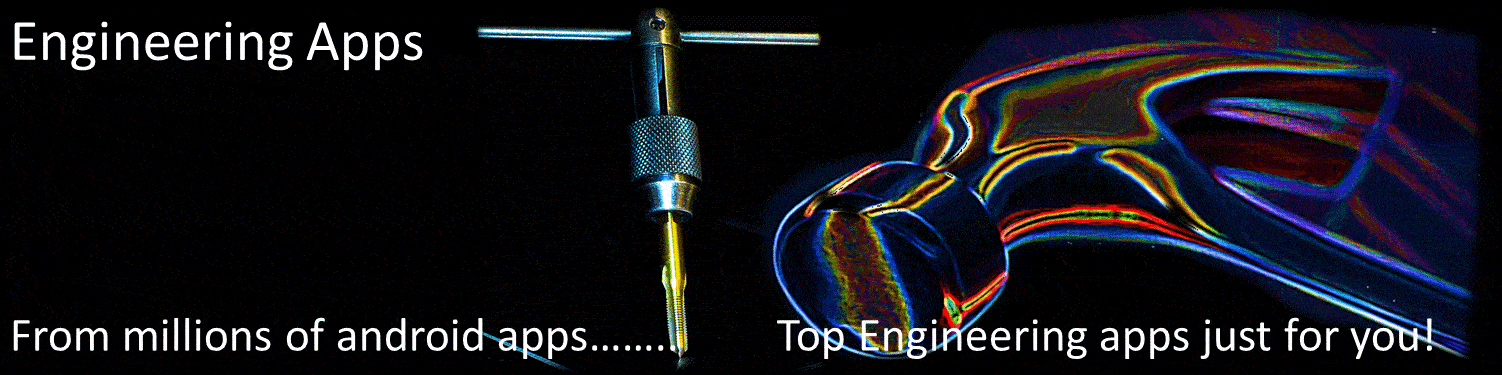••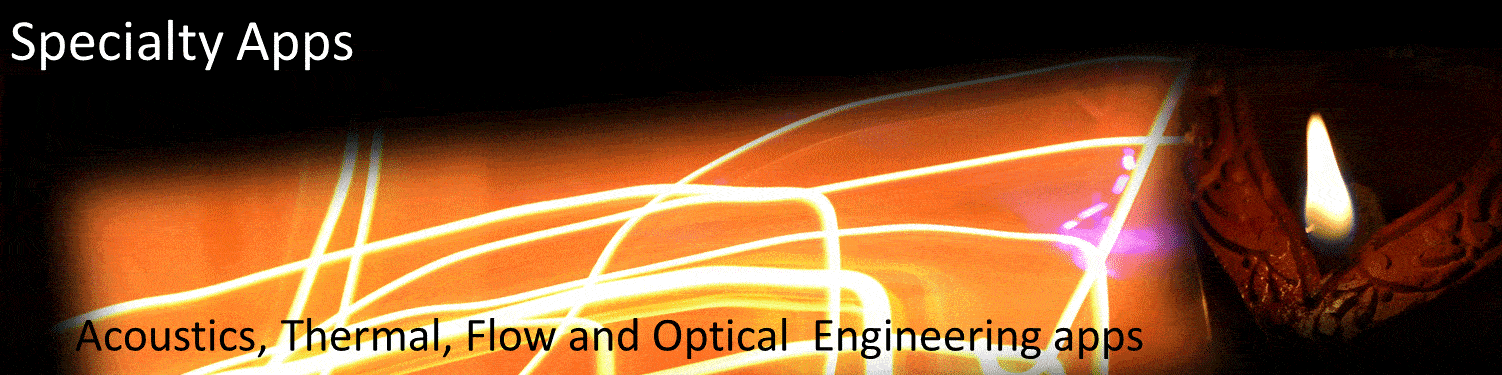•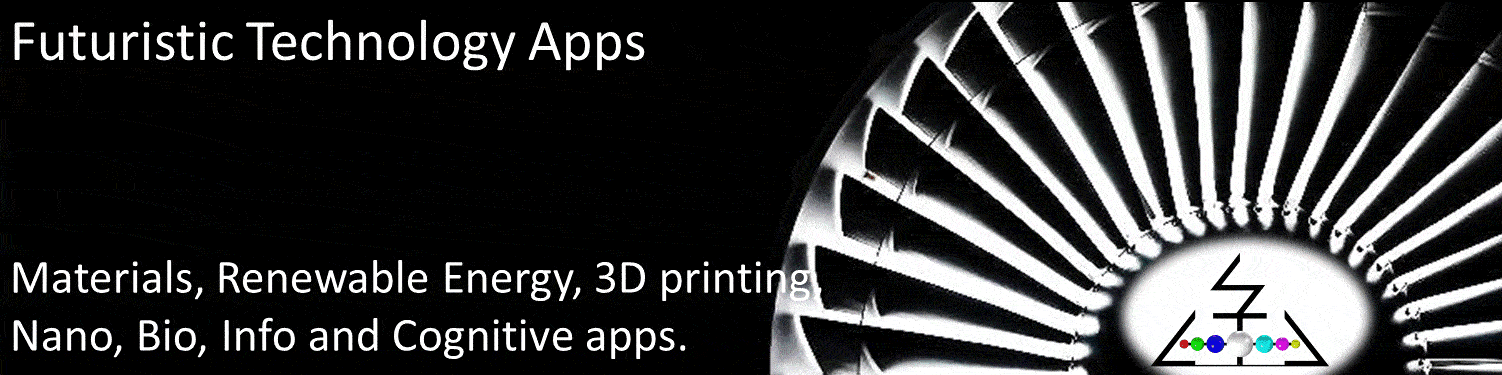•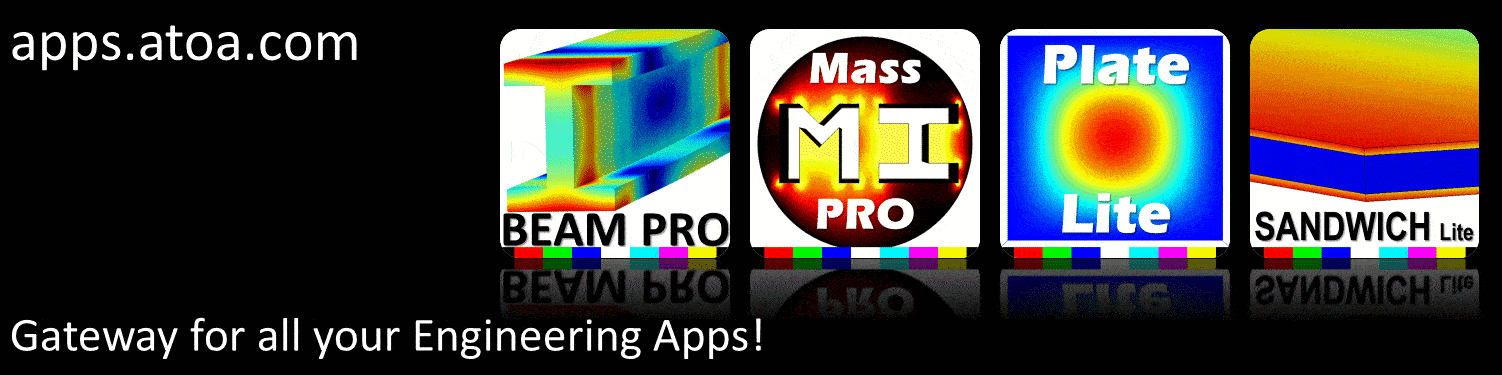•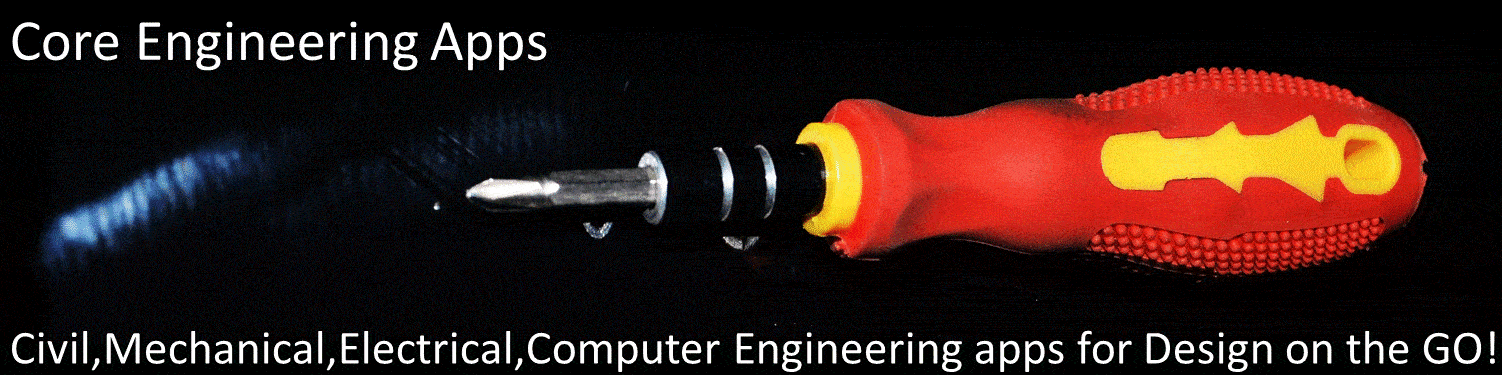### Mass moment of Inertia PRO

CALCULATE 2nd MI

Using this tool you can draw any arbitrary shape and calculate mass moment of inertia properties. These are useful for structural mechanics and dynamics performance prediction .Mass moment of inertia is critical for mechanical, rotation, dynamics performance prediction.

##### A T O A   A P P S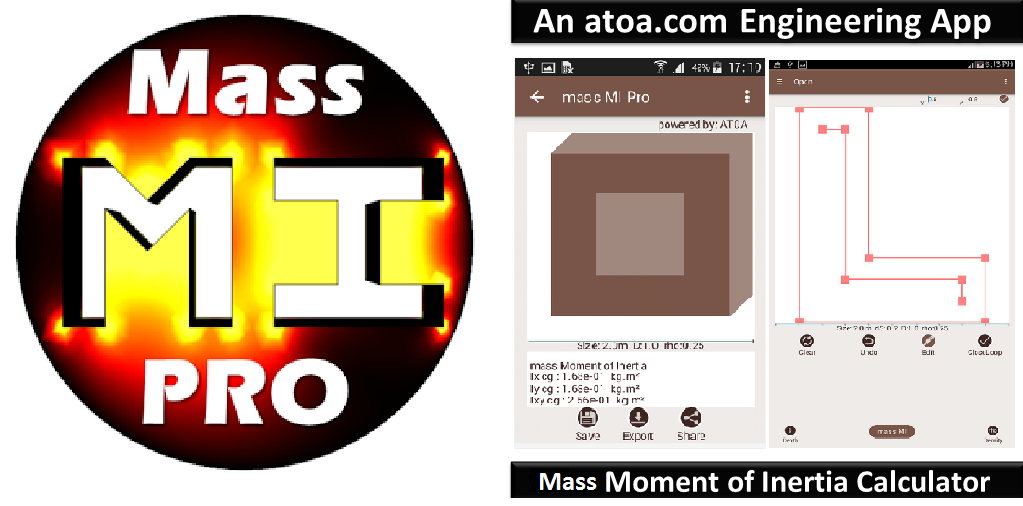##### A P P L I C A T I O N   D E S C R I P T I O N

This tool helps to draw any open, closed, cut, holed 2D cross section, extrude to 3D geometry and then calculate mass moment of inertia properties. These are useful for structural mechanics and dynamics performance prediction. Mass moment of inertia is critical for mechanical, rotation, dynamics performance prediction. Mass moment of inertia may also be termed as rotational inertia, polar moment of inertia, or the angular mass.MI properties for a standard shapes can be obtained from a list of table or standard formula. But for irregular shapes and nonstandard sizes, the mass properties are not readily available. This tool allows you to draw any shape, extrude and then calculate geometric and inertial properties for any arbitrary shape. Geometric and MI properties of any regular/ standard shape can also be predicted, by taping the cross section.

Draw non intersecting outer by tapping the vertex of your 2D cross section. You can also create a hole or cut by tapping the vertex of your cut or hole. A unit depth of extrusion and unit density is assumed for mass property prediction. Then click "mass MI" to calculate the mass properties. The default drawing window size is 2 m and snap size is 0.02 m. You can change by tapping the settings. You can also edit the X,Y coordinates of the vertex for more accurate numeric input. Select your convenient Units. Set the window size to the maximum size of your 2D shape. This size can be set to any size from nano meter (10-9) to giga meter (10+9). Mass MI Lite tool supports any shape bounded by non-intersecting outer straight line segments. Draw by tapping the vertex in clock wise or counter clock wise pattern. Avoid crossing of the boundary. The end point can be closed by tapping close end. Clear, Undo, Edit and CloseLoop buttons are useful to start with any shape, edit the vertex to change shapes, add lines, or move lines and or close the boundary.

The mass moment of inertia is a measure of the 'efficiency' of any shape to resist rotation. Mass MI is the property of a rigid body that determines the torque needed for a desired angular acceleration about an axis of rotation. Moment of inertia depends on the shape of the body and the axes of rotation.The geometry of any arbitrary shape can be defined using non intersecting external boundary lines.

The area bounded by these lines is calculated using area integration method. The external boundary is represented by linear piece-wise straight lines. A general formula for the moments of arbitrary shape bounded by straight lines is derived leveraging Green's theorem for transforming domain to boundary integrals. This formula is implemented as an algorithm for computing inertial properties.

The tool prediction results for standard shapes are compared with Formulas from text book, Numerical Integration solution using Industrial FEA solvers. For straight lined boundary based geometry the prediction accuracy is very high. For curved boundary the accuracy can be improved by increasing the number of vertex. But for arbitrary shapes such as corroded or damaged members, custom built sections, 3D printed shapes, complex designs, Mass MI LITE is the only tool which will help to predict mass properties.

The number of vertices is limited to 14 and functionalities (open, save, import, export, share) are limited to 5 in this free version. The Pro version will enable you do draw any shape with virtually unlimited vertices and functionalities. The Pro version is fully functional with A, V, m, Ixx, Iyy, Ixy Properties, XY coordinates, Holes, composite sections, save, data import/export, suggestions and share functionalities.

S C R E E N   S H O T S

##### Loading ...DownloadOur mission is to make " Engineering for all" to "Design on the GO!"